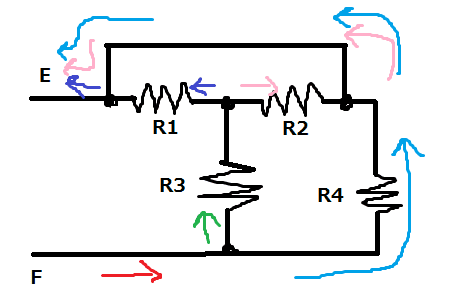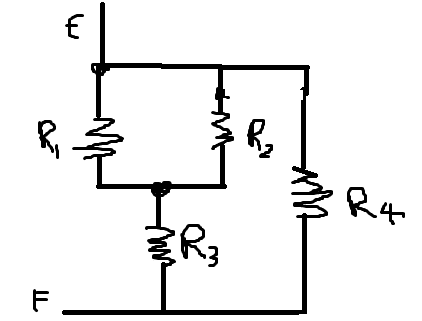# Finding the equivalent resistance of this circuit.

## Homework Statement

I got this on a quiz today - Find the equivalent resistance between E and F.

Now, the problem with this question is that I get TWO different answers when I trace from:

1. e to f
2. f to e

I know one of them is definitely wrong (or maybe both are?), but I don't know which.## The Attempt at a Solution

Now, if I go from E to F, I get:

1. Current goes through short circuit, then splits between two paths. so R4//(R2+R3)

If I go from F to E (which I've drawn in colors), I get:

2. Current splits between R3 and R4. Then following R3, it splits into R1 and R2. R4 then follows through the short circuit back to E, so R4//(R3+R1//R2)

In the end I had to pick one answer so I randomly chose 2 for arbitrary reasons. But what's the way to go about this problem, and which one is correct? (IF one of them is?)

CWatters
Homework Helper
Gold Member
Don't rush to write equations. Look carefully at the circuit to see if it can be simplified.

Hint: Take a good look at R4. Once you have seen that one take a look at R2. It's actually in parallel with another resistor.

Don't rush to write equations. Look carefully at the circuit to see if it can be simplified.

Hint: Take a good look at R4. Once you have seen that one take a look at R2. It's actually in parallel with another resistor.

One sec.

Last edited:
SammyS
Staff Emeritus
Homework Helper
Gold Member

## Homework Statement

I got this on a quiz today - Find the equivalent resistance between E and F.

Now, the problem with this question is that I get TWO different answers when I trace from:

1. e to f
2. f to e

I know one of them is definitely wrong (or maybe both are?), but I don't know which.

## The Attempt at a Solution

Now, if I go from E to F, I get:

1. Current goes through short circuit, then splits between two paths. so R4//(R2+R3)

If I go from F to E (which I've drawn in colors), I get:

2. Current splits between R3 and R4. Then following R3, it splits into R1 and R2. R4 then follows through the short circuit back to E, so R4//(R3+R1//R2)

In the end I had to pick one answer so I randomly chose 2 for arbitrary reasons. But what's the way to go about this problem, and which one is correct? (IF one of them is?)

It would be much easier to discuss this circuit if you would label all of the nodes (with letters).

It would be much easier to discuss this circuit if you would label all of the nodes (with letters).

Because there are nodes in between all resistors, it is almost impossible simplify the circuit using parallel/series connection. Should I redraw the circuit in some way?

Last edited:
SammyS
Staff Emeritus
Homework Helper
Gold Member
Because there are nodes in between all resistors, it is almost impossible simplify the circuit using parallel/series connection. Should I redraw the circuit in some way?
No. It's perfectly possible, and quite easy, to analyse this as series/parallel.

The entire "shorting wire" along with the node right of R2, the node left of R1, and point E can all be collapsed into one node.

R4 connects E & F, so it's in parallel with all the rest of the circuit.

Any current flowing into and then out of R3 (from F) then either goes through R1 or goes through R2 after which it goes to E.

No. It's perfectly possible, and quite easy, to analyse this as series/parallel.

The entire "shorting wire" along with the node right of R2, the node left of R1, and point E can all be collapsed into one node.

R4 connects E & F, so it's in parallel with all the rest of the circuit.

Any current flowing into and then out of R3 (from F) then either goes through R1 or goes through R2 after which it goes to E.

Hang on. I see that R4 directly connects E and F (due to the short circuit), but how can any current going from E to F passing through R3 NOT also pass through some other resistor?

berkeman
Mentor
Because there are nodes in between all resistors, it is almost impossible simplify the circuit using parallel/series connection. Should I redraw the circuit in some way?

That's what I did to help me see how the resistors are connected. And as stated by others, it can be solved with series/parallel simplifications.

Try drawing it this way: Make R1, R2, and R4 all be vertical, and level with each other. Show R3 vertical below R1 and R2, about mid-way between them. Then show the connecting wires that make the same connections as shown in the original drawing. You should now see what you can combine: first a parallel combination, then a series one, and then a final parallel combination...

SammyS
Staff Emeritus
Homework Helper
Gold Member
Hang on. I see that R4 directly connects E and F (due to the short circuit), but how can any current going from E to F passing through R3 NOT also pass through some other resistor?
I think that I said that it does. Going from F to E: After passing through R3, it passes through either R1 or R2 then goes to E.

Sorry Sammy, I accidentally read "neither"

I thank you both.SammyS
Staff Emeritus
Homework Helper
Gold Member
Sorry Sammy, I accidentally read "neither"

I thank you both.Perfect !

It does give a pretty messy answer!

Thank you for your help! But that would mean my number 2 answer is correct, is it not?

SammyS
Staff Emeritus
Homework Helper
Gold Member
Thank you for your help! But that would mean my number 2 answer is correct, is it not?
R4//(R3+R1//R2)
which doesn't make any sense.

... or does RA//RB meant that RA is in parallel with RB, meaning that 1/(RA//RB) = 1/RA + 1/RB ?

If that's the case, you probably need another set of parentheses:

R4//(R3+(R1//R2)) .

Wouldn't, || or ‖ be more obvious than // ?